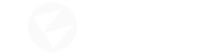# Random Vector3 position!

Hi,
How to get a random vector3 position? I could not find random function in C#. So below is the code which I found online -

``````public double RandomNumber(int minimum, int maximum)
{
Random random = new Random();
return random.Next(maximum - minimum);
}
``````

And here is how I am using it -

``````Vector3 Direction()
{
targetPos = new Vector3(targetPos.X + RandomNumber(-factor, factor), targetPos.Y + RandomNumber(-factor,  factor), targetPos.Z + RandomNumber(-factor,  factor));
Vector3 direction = targetPos - cameraHead.Position;
return direction.Normalized;
}
``````

It creates a random position but just in a straight line. See the picture below.

That above Direction function is taken from one of my unity game project and it works nicely in unity. Unity has its own Random function. Any suggestion will be appreciated.

The `System.Random` class is unnecessarily convoluted to use, although it wasn’t designed with game development in mind. It would be nice if Flax implemented their own Random class like Unity.

To answer your question, I think I solved the issue by using the code below:

``````Vector3 Direction()
{
int factor = 2;
Vector3 targetPos = Actor.Position;

Random random = new Random(ID.GetHashCode() + DateTime.Now.Millisecond);
targetPos.X += (float)random.Next(-factor, factor);
targetPos.Y += (float)random.Next(-factor, factor);
targetPos.Z += (float)random.Next(-factor, factor);

Vector3 direction = targetPos - Actor.Position;
return direction.Normalized;
}
``````

I don’t know for sure why this works, but I have a few theories:

`Random`'s default seed is time dependent, so if the original code is executed across multiple Actors at the same time, the same seed is used. This adds the Actor’s unique ID into the mix so that each random number is different for each Actor, regardless of the time.

Creating a new `Random` object for each random number also seems to mess up the RNG. Using the same object worked for me.

You should be able to use `FlaxEngine.Utilities`, which has some pretty useful random methods

It’s probably useful enough to warrant at least a little note somewhere in the documentation. Pull requests are always super appreciated.

About the `Random` object, yeah, the way to go is to create it once and reuse that object.

3 Likes

Thanks for the tip!

Thanks Spectrix. This method works nicely with auto and single shot guns. But seems like it has a little problem with shotgun. But still works. It would be nice if Flax implements its own random method like unity. Thank you.

1 Like